一文带你认识队列数据结构前言队列介绍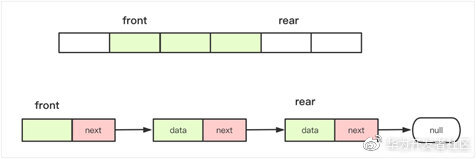​实现方法：

• MyCircularQueue(k): 构造器，设置队列长度为 k 。

• Front: 从队首获取元素。如果队列为空，返回 -1 。

• Rear: 获取队尾元素。如果队列为空，返回 -1 。

• enQueue(value): 向循环队列插入一个元素。如果成功插入则返回真。

• deQueue(): 从循环队列中删除一个元素。如果成功删除则返回真。

• isEmpty(): 检查循环队列是否为空。

• isFull(): 检查循环队列是否已满。

普通队列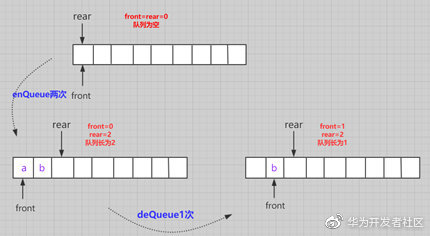​在这个普通队列一些操作需要注意的有：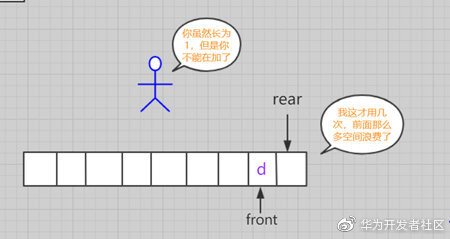循环队列(数组实现)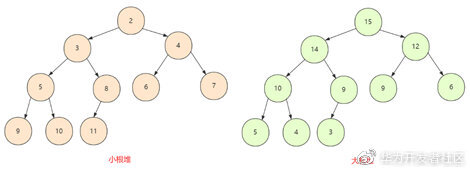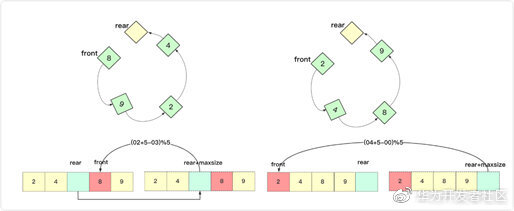​这里面有几个大家需要注意的，就是指标相加如果遇到最后需要转到头的话。可以判断是否到数组末尾位置。也可以直接+1 求余。其中 maxsize 是数组实际大小。

public class MyCircularQueue {    private int data[];// 数组容器    private int front;// 头    private int rear;// 尾    private int maxsize;// 最大长度    public MyCircularQueue(int k) {        data = new int[k+1];        front = 0;        rear = 0;        maxsize = k+1;    }
public boolean enQueue(int value) { if (isFull()) return false; else { data[rear] = value; rear=(rear + 1) % maxsize; } return true; }
public boolean deQueue() { if (isEmpty()) return false; else { front=(front+1)%maxsize; } return true; }
public int Front() { if(isEmpty()) return -1; return data[front]; }
public int Rear() { if(isEmpty()) return -1; return data[(rear-1+maxsize)%maxsize]; }
public boolean isEmpty() { return rear == front; }
public boolean isFull() { return (rear + 1) % maxsize == front; }}

循环队列(链表实现)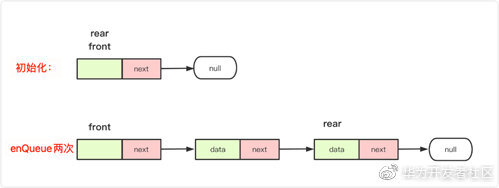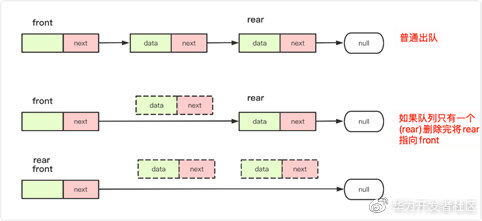public class MyCircularQueue{     class node {        int data;// 节点的结果        node next;// 下一个连接的节点        public node() {}        public node(int data) {            this.data = data;        }    }    node front;//相当于head 带头节点的    node rear;//相当于tail/end    int maxsize;//最大长度    int len=0;    public MyCircularQueue(int k) {        front=new node(0);        rear=front;        maxsize=k;        len=0;    }    public boolean enQueue(int value)  {        if (isFull())            return  false;        else {            node va=new node(value);            rear.next=va;            rear=va;            len++;        }        return  true;    }    public boolean deQueue() {        if (isEmpty())            return false;        else {            front.next=front.next.next;            len--;            //注意 如果被删完 需要将rear指向front            if(len==0)                rear=front;        }        return  true;    }
public int Front() { if(isEmpty()) return -1; return front.next.data; }
public int Rear() { if(isEmpty()) return -1; return rear.data; }
public boolean isEmpty() { return len==0; //return rear == front; }
public boolean isFull() { return len==maxsize; } }

双向队列(加餐)

• MyCircularDeque(k)：构造函数,双端队列的大小为 k。

• insertFront()：将一个元素添加到双端队列头部。 如果操作成功返回 true。

• insertLast()：将一个元素添加到双端队列尾部。如果操作成功返回 true。

• deleteFront()：从双端队列头部删除一个元素。 如果操作成功返回 true。

• deleteLast()：从双端队列尾部删除一个元素。如果操作成功返回 true。

• getFront()：从双端队列头部获得一个元素。如果双端队列为空，返回 -1。

• getRear()：获得双端队列的最后一个元素。 如果双端队列为空，返回 -1。

• isEmpty()：检查双端队列是否为空。

• isFull()：检查双端队列是否满了。

public class MyCircularDeque {    private int data[];// 数组容器    private int front;// 头    private int rear;// 尾    private int maxsize;// 最大长度    /*初始化 最大大小为k */    public MyCircularDeque(int k) {        data = new int[k+1];        front = 0;        rear = 0;        maxsize = k+1;    }
/** 头部插入 */ public boolean insertFront(int value) { if(isFull()) return false; else { front=(front+maxsize-1)%maxsize; data[front]=value; } return true; }
/** 尾部插入 */ public boolean insertLast(int value) { if(isFull()) return false; else{ data[rear]=value; rear=(rear+1)%maxsize; } return true; }
/** 正常头部删除 */ public boolean deleteFront() { if (isEmpty()) return false; else { front=(front+1)%maxsize; } return true; }
/** 尾部删除 */ public boolean deleteLast() { if(isEmpty()) return false; else { rear=(rear+maxsize-1)%maxsize; } return true; }
/** Get the front item */ public int getFront() { if(isEmpty()) return -1; return data[front]; }
/** Get the last item from the deque. */ public int getRear() { if(isEmpty()) return -1; return data[(rear-1+maxsize)%maxsize]; }
/** Checks whether the circular deque is empty or not. */ public boolean isEmpty() { return front==rear; }
/** Checks whether the circular deque is full or not. */ public boolean isFull() { return (rear+1)%maxsize==front; }}

总结评论